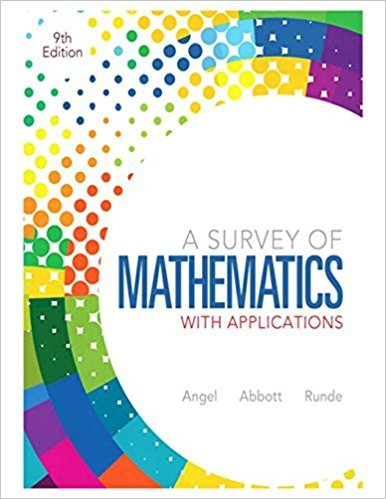×
×

# Solutions for Chapter 7.1: Systems of Linear Equations and Inequalities## Full solutions for A Survey of Mathematics with Applications | 9th Edition

ISBN: 9780321759665Solutions for Chapter 7.1: Systems of Linear Equations and Inequalities

Solutions for Chapter 7.1
4 5 0 373 Reviews
20
5
##### ISBN: 9780321759665

Chapter 7.1: Systems of Linear Equations and Inequalities includes 61 full step-by-step solutions. This textbook survival guide was created for the textbook: A Survey of Mathematics with Applications, edition: 9. A Survey of Mathematics with Applications was written by and is associated to the ISBN: 9780321759665. This expansive textbook survival guide covers the following chapters and their solutions. Since 61 problems in chapter 7.1: Systems of Linear Equations and Inequalities have been answered, more than 74066 students have viewed full step-by-step solutions from this chapter.

Key Math Terms and definitions covered in this textbook
• Affine transformation

Tv = Av + Vo = linear transformation plus shift.

• Characteristic equation det(A - AI) = O.

The n roots are the eigenvalues of A.

• Cholesky factorization

A = CTC = (L.J]))(L.J]))T for positive definite A.

• Column picture of Ax = b.

The vector b becomes a combination of the columns of A. The system is solvable only when b is in the column space C (A).

• Companion matrix.

Put CI, ... ,Cn in row n and put n - 1 ones just above the main diagonal. Then det(A - AI) = ±(CI + c2A + C3A 2 + .•. + cnA n-l - An).

• Covariance matrix:E.

When random variables Xi have mean = average value = 0, their covariances "'£ ij are the averages of XiX j. With means Xi, the matrix :E = mean of (x - x) (x - x) T is positive (semi)definite; :E is diagonal if the Xi are independent.

• Cyclic shift

S. Permutation with S21 = 1, S32 = 1, ... , finally SIn = 1. Its eigenvalues are the nth roots e2lrik/n of 1; eigenvectors are columns of the Fourier matrix F.

• Diagonal matrix D.

dij = 0 if i #- j. Block-diagonal: zero outside square blocks Du.

• Dimension of vector space

dim(V) = number of vectors in any basis for V.

• Echelon matrix U.

The first nonzero entry (the pivot) in each row comes in a later column than the pivot in the previous row. All zero rows come last.

• Factorization

A = L U. If elimination takes A to U without row exchanges, then the lower triangular L with multipliers eij (and eii = 1) brings U back to A.

• Left nullspace N (AT).

Nullspace of AT = "left nullspace" of A because y T A = OT.

• Lucas numbers

Ln = 2,J, 3, 4, ... satisfy Ln = L n- l +Ln- 2 = A1 +A~, with AI, A2 = (1 ± -/5)/2 from the Fibonacci matrix U~]' Compare Lo = 2 with Fo = O.

• Matrix multiplication AB.

The i, j entry of AB is (row i of A)·(column j of B) = L aikbkj. By columns: Column j of AB = A times column j of B. By rows: row i of A multiplies B. Columns times rows: AB = sum of (column k)(row k). All these equivalent definitions come from the rule that A B times x equals A times B x .

• Nullspace N (A)

= All solutions to Ax = O. Dimension n - r = (# columns) - rank.

• Reduced row echelon form R = rref(A).

Pivots = 1; zeros above and below pivots; the r nonzero rows of R give a basis for the row space of A.

• Row space C (AT) = all combinations of rows of A.

Column vectors by convention.

• Similar matrices A and B.

Every B = M-I AM has the same eigenvalues as A.

• Simplex method for linear programming.

The minimum cost vector x * is found by moving from comer to lower cost comer along the edges of the feasible set (where the constraints Ax = b and x > 0 are satisfied). Minimum cost at a comer!

• Spectrum of A = the set of eigenvalues {A I, ... , An}.

Spectral radius = max of IAi I.

×